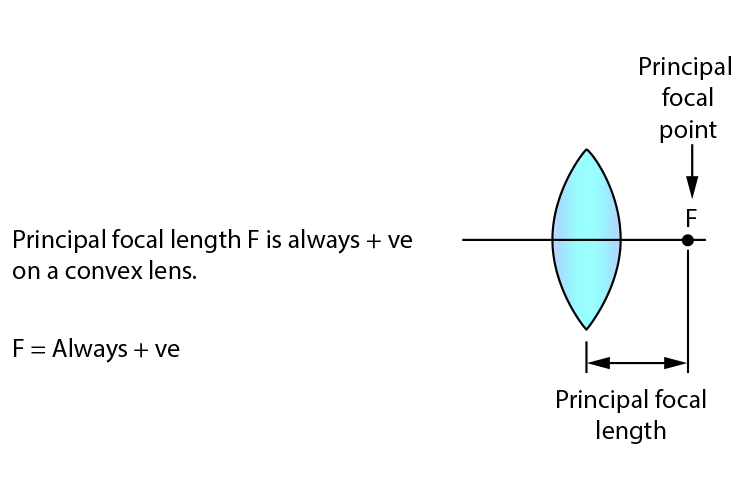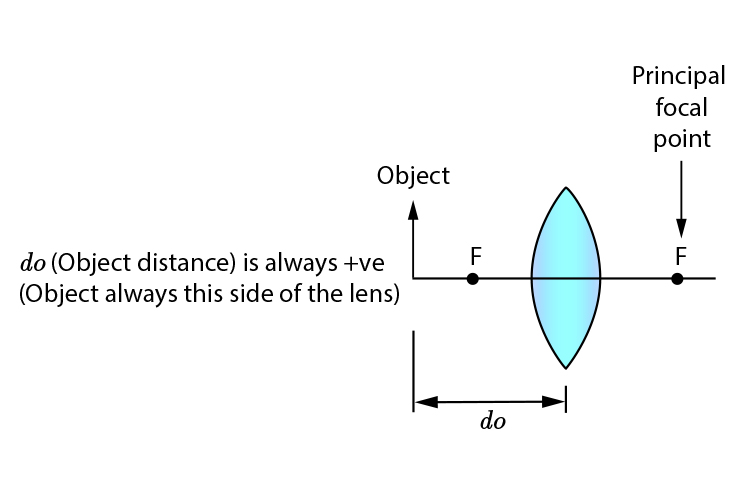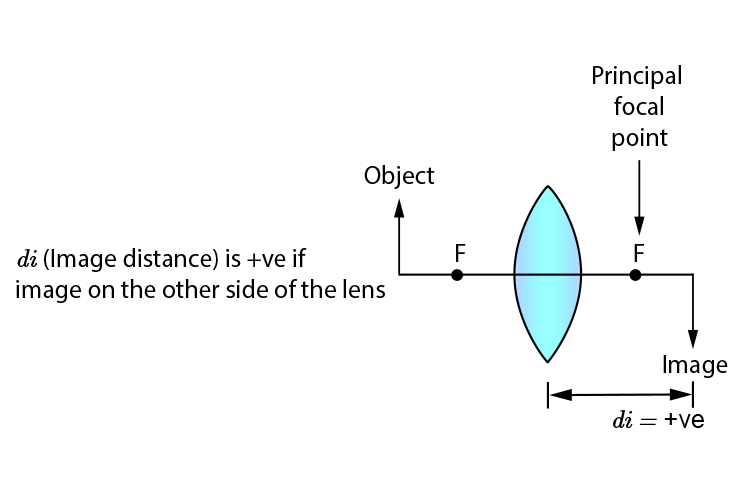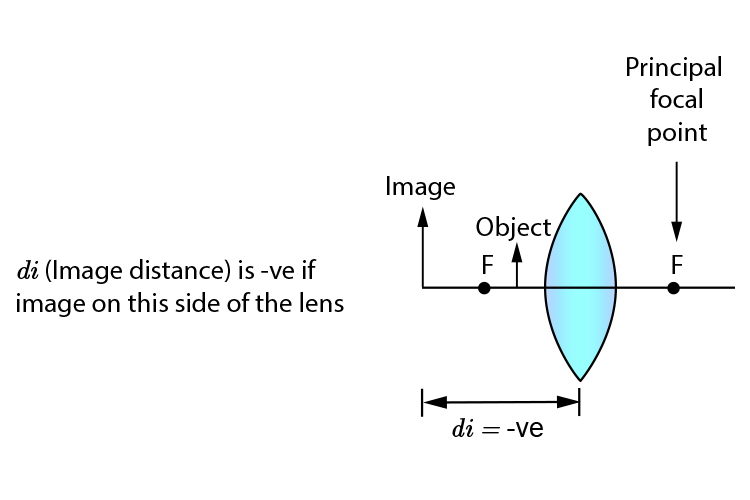# Convex lenses and the lens equation

We can use the lens equation that we have previously seen to find the principal focal point.

In an exam, the lens equation may be used in a whole host of questions.

As we know, the lens equation is:

Lens equation
IF I DO I DIE

I/F=I/(do)+I/(di)

1/F=1/(do)+1/(di)

Where

 F = Principal focal length do = Object distance from centre of convex lens di = Image distance from centre of convex lens

NOTE: This works for lenses that are thin but the thicker a lens gets the less accurate the equation becomes. But for general purposes and basic exams, this equation is normally used.

You will need to be aware of what is positive and what is negative.

## Convex single lens## Summary

If you use the lens equation for convex lenses ALL NUMBERS are +ve except for one number, di, which is negative if the image is on the object side.